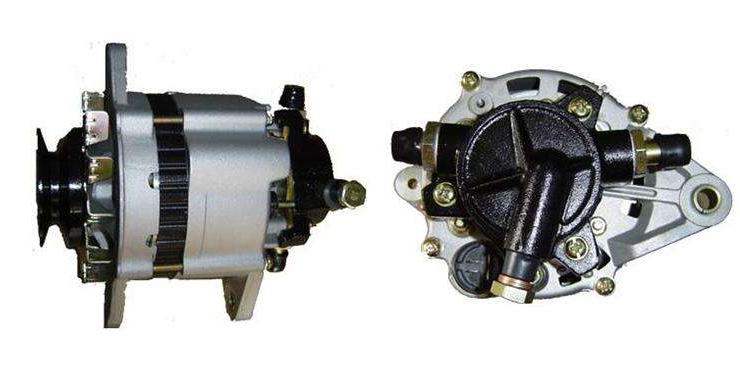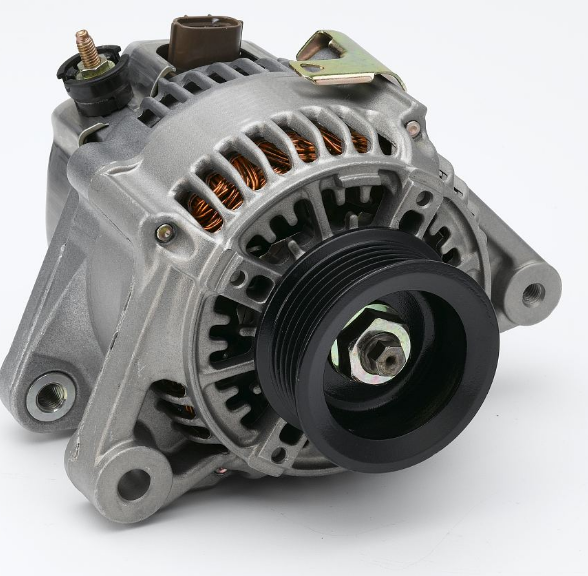About Us

# What is the difference between an ac generator and a dc generator?

What is the difference between an ac generator and a dc generator? The electricity produced by a dc generator is alternating current (ac), while a dc generator is alternating current (ac), which is then converted to dc by an electronic rectifier or commutator.1. Similarities

(1) same structure: the structure of alternator and dc motor is composed of stator, rotor, iron core, coil, magnetic pole, brush, etc.

(2) the electromagnetic application is the same: both are the application of electromagnetism and electromagnetism.

(3) conversion use the same: asynchronous motor, as long as the prime mover (such as water turbine, diesel engine, etc.) drag, so that the rotor speed n is higher than the rotating magnetic field of synchronous speed n1, even if S= negative slip, plus the excitation current, it becomes an ac asynchronous generator; Dc motor, if the dynamic part of its change to external power rotation, its armature winding and excitation winding end have dc voltage, this shows that dc motor can also be used as a dc generator.(4) operation maintenance: in the operation of all needs to check its operation, sound and vibration is normal, the ventilation is good, bearing oil, adequacy of stator winding and the temperature of each part is normal, the rotor brush on the presence of abnormal spark, voltage and current is within the scope of the rating, chassis ground zero are in good condition and etc.

2. Differences

(1) working principle: alternator is made according to the principle of electromagnetic induction, that is, whether it's conductor movement (coil), or magnetic activity, as long as there is relative motion between a conductor (coil) and magnetic field, the conductor cutting lines (coil), will produce electromotive force in the conductor (coil), which moved to electric power; Dc motor is based on the current carrying conductor (coil) in the magnetic field force and movement of the principle of production, that is, through the electricity can move called electric.

(2) the structure is different: each end of the alternator coil is connected with a copper ring (slip-ring), that is, a brush; The dc motor coils are each connected to a copper half ring called a commutator, which plays a commutating role on the current of each winding, making alternating current into direct current.

(3) direction determination is different: the direction of the induction electromotive force of the alternator is determined by using the right hand rule; The direction of rotation of the dc motor is determined by the left hand rule.

(4) the conversion of energy is different: what the alternator inputs is mechanical energy, and what it outputs is electrical energy; A rotating motor that converts mechanical energy into electrical energy; The dc motor is the input of electrical energy, the output is mechanical energy, the electrical energy into the mechanical energy of the motor.